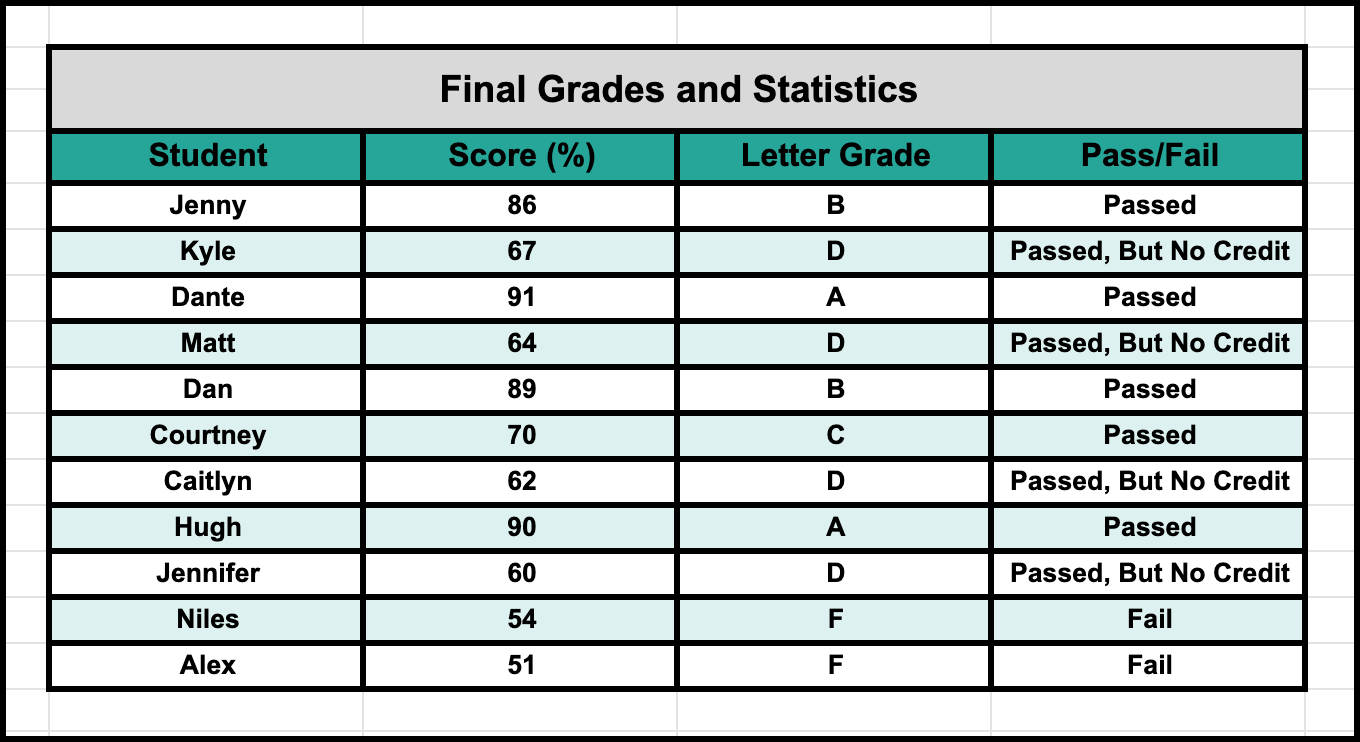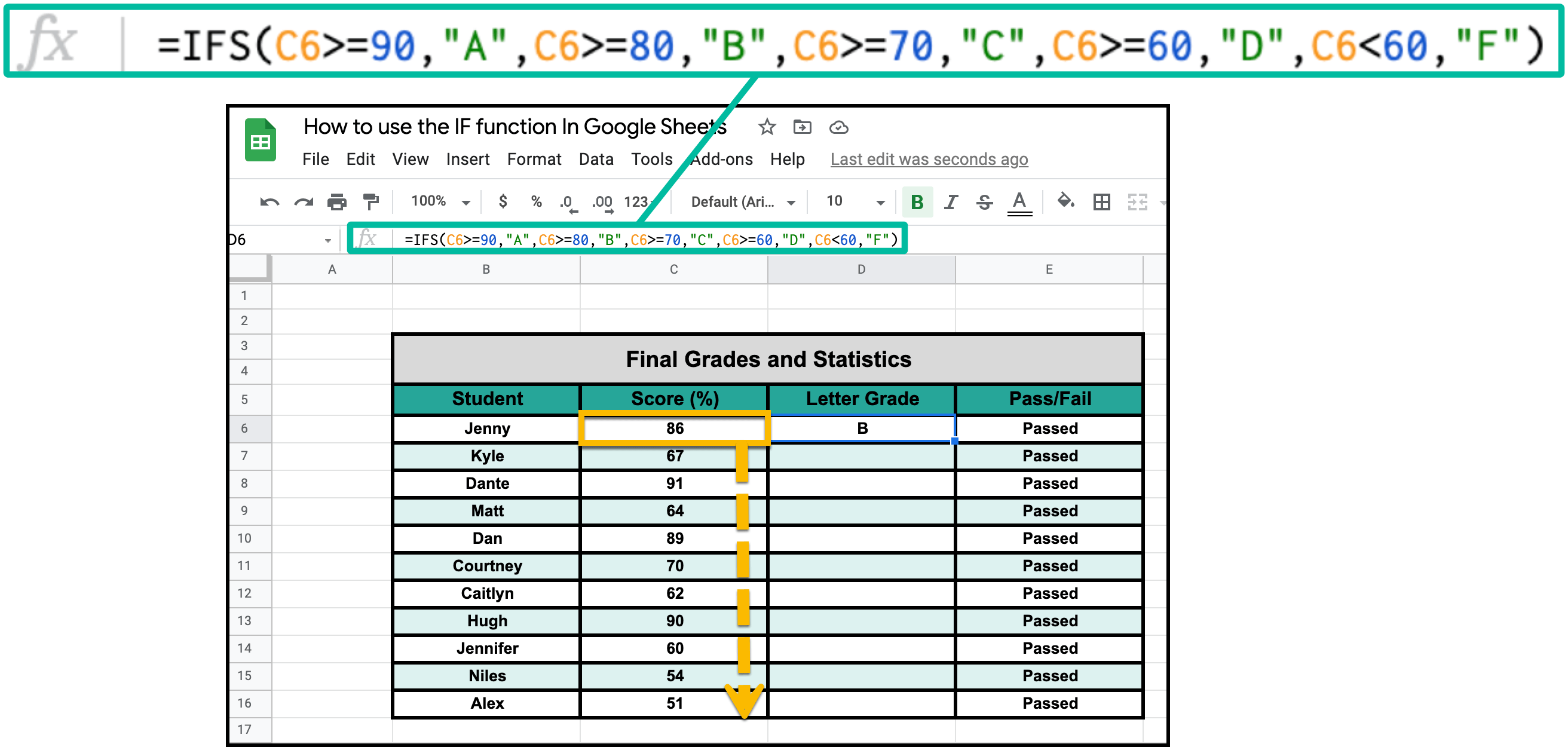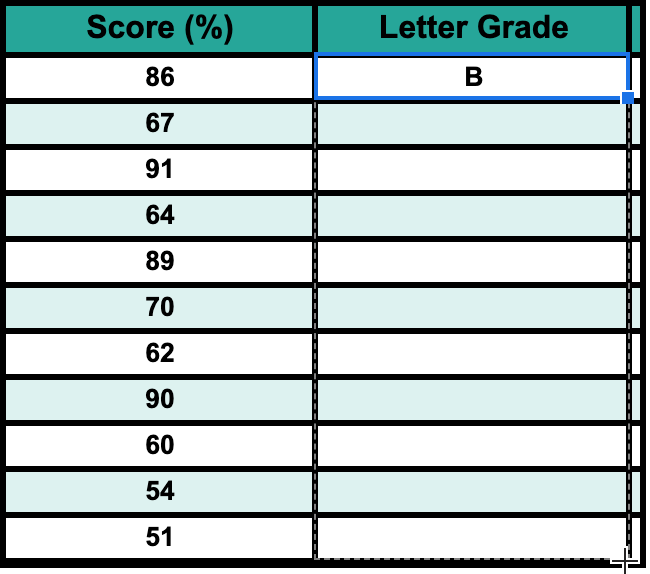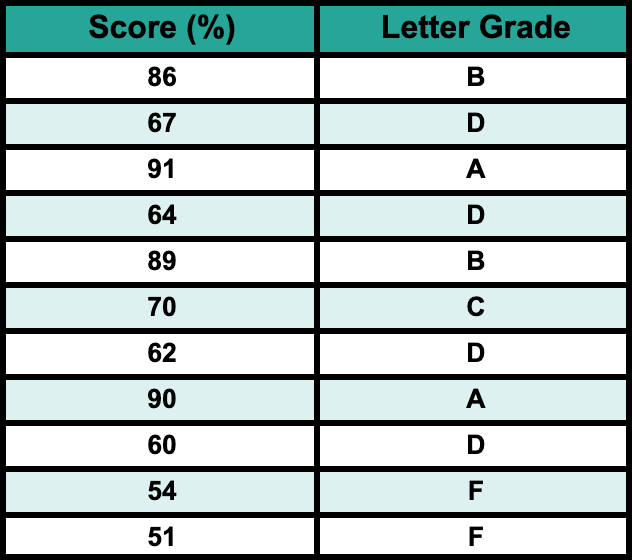# How to Use the IFS Function in Google SheetsIn google sheets, the IFS, much like the IF function, is used to conditionally format your data according to various criteria and multiple conditions. While the IF function works best under "A or B" scenarios, IFS lets you add as much criteria as is needed in a much simpler fashion.

Let's Walk through google sheet's version of IFS() together.

## Step #1: =IFS()First, you will need to understand how it works. For this example, I want to calculate the letter grade for the students in my imaginary class. As is the case with most classes:

90% =A

80% =B

70% =C

60% =D

Below 60% =F

As such, we need to write a function that expresses that. While it is possible to do so with IF, it is very complicated, and IFS makes it way easier to understand. The function looks something like this:

=IFS(C6>=90,"A",C6>=80,"B",C6>=70,"C",C6>=60,"D",C6<60,"F")

## Step #2: Let Sheets "Rationalize"Here is how Google Sheets "rationalizes" the data, or at least how I like to think that it does:

1.  Is the number greater than or equal to 90? If yes, post "A" and end the scan, otherwise assume it's below 90 and go to step 2.
2. Is the number greater than or equal to 80? If yes, post "B" and end the scan, otherwise assume it's less than 80 and go to step 3.
3. Is the number greater than or equal to 70? If yes, post "C" and end the scan, otherwise assume it's less than 70 and go to step 4.
4. Is the number greater than or equal to 60? If yes, post "D" and end the scan, otherwise assume its less than 60 and move on to step 5
5. Is the number greater than or equal to 60? If yes, post "F" and end the scan.

From here, you have a set algorithm, a plan of attack for Google Sheets to follow more powerful than some simple True/False statement.